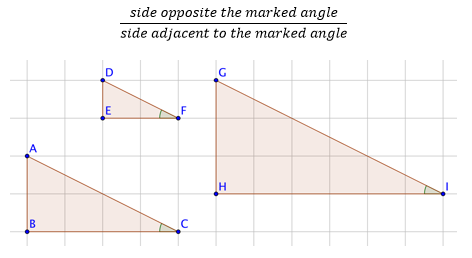# Right Triangles and Trigonometry

## Objective

Describe and calculate tangent in right triangles. Describe how the value of tangent changes as the angle measure approaches 0°, 45°, and 90°.

## Common Core Standards

### Core Standards

?

• G.SRT.C.6 — Understand that by similarity, side ratios in right triangles are properties of the angles in the triangle, leading to definitions of trigonometric ratios for acute angles.

• G.SRT.C.7 — Explain and use the relationship between the sine and cosine of complementary angles.

?

• G.CO.C.10

## Criteria for Success

?

1. Calculate the ratio of the opposite side to the adjacent side of a 30° angle in similar right triangles.
2. Define the tangent of any given angle in a right triangle as the ratio of the side length of the opposite side to that angle over the adjacent side (TOH).
3. Describe that the tangent of any given angle is equal across all triangles with the same angle measures, extending from the angle-angle criterion for similarity.
4. Calculate the tangent of any degree measure in a triangle using a scientific or graphing calculator.
5. Identify and memorize the tangent for common angle measures of 0°, 30°, 45°, 60°, and 90°.
6. Explain why the tangent of any 90° angle is undefined.
7. Determine the relationship between sine, cosine, and tangent, and write the definition of tangent in terms of sine and cosine.

## Tips for Teachers

?

Memorization of the tangent for common angle measures is not required by the Common Core standards. However, if students have these values memorized, they will be better able to access some Algebra 2 and AP Calculus material.

## Anchor Problems

?

### Problem 1

Below is a set of similar right triangles. Find the ratio of the side lengths within each triangle that describe the side opposite the marked angle divided by the side adjacent to the marked angle.### Problem 2

What is the tangent of 0°, 45°, 60°, and 90°?   Describe why the tangent of 90° is undefined.

### Problem 3

For a right triangle, is the following statement always, sometimes, or never true?

${\mathrm{tan}\theta=\frac{\mathrm{sin}\theta}{\mathrm{cos}\theta}}$

#### References

EngageNY Mathematics Geometry > Module 2 > Topic E > Lesson 30Example 2

Geometry > Module 2 > Topic E > Lesson 30 of the New York State Common Core Mathematics Curriculum from EngageNY and Great Minds. © 2015 Great Minds. Licensed by EngageNY of the New York State Education Department under the CC BY-NC-SA 3.0 US license. Accessed Dec. 2, 2016, 5:15 p.m..

Modified by Fishtank Learning, Inc.

## Problem Set

?

The following resources include problems and activities aligned to the objective of the lesson that can be used to create your own problem set.

• Include problems where students need to calculate the tangent of non-special right triangles and then verify that the tangent is the same for any triangles that are similar.
• Include problems where students need to use the tangent and one actual side length to calculate the other side lengths of the triangle.

If ${\mathrm{sin}\theta=\frac{\sqrt5}{5}}$, find ${\mathrm{cos}\theta}$ and ${\mathrm{tan}\theta}$.